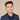# How To Find Index Of List Item In Swift

To find the index of a list item, you can use the `firstIndex` or `lastIndex` function:

``````let arr = [1, 2, 3, 4, 1]

let firstIndex = arr.firstIndex(of: 1) // 0
let lastIndex = arr.lastIndex(of: 1) // 4``````

If you have an class or want to check a condition, you can use the `where` instead of `of`.

``````let arr = [1, 2, 3, 4, 1]

let firstIndex = arr.firstIndex(where: { \$0 % 2 == 0} )
let lastIndex = arr.lastIndex(where: { \$0 % 2 == 0} )
print(firstIndex)
print(lastIndex)``````

The above code checks for even numbers and prints:

``````Optional(1)
Optional(3)``````

Note that `firstIndex` and `lastIndex` return optionals and can be nil if the element is not found.

You could do the same with a custom object, for example:

``let firstIndex = personArray.firstIndex(\$0.name == "Eddy")``

## Get All Indexes Of Filtered Item In Array

To do this, it’s best to create custom array extension:

``````extension Array where Element: Equatable {
func indexes(of element: Element) -> [Int] {
return self.enumerated().filter({ element == \$0.element }).map({ \$0.offset })
}
}``````

Then you can call it like so:

``````let arr = [1, 2, 3, 4, 1]
arr.indexes(of: 1)
print(arr)``````

This prints:

``[0, 4]``
If you liked this post and want to learn more, check out The Complete iOS Developer Bootcamp. Speed up your learning curve - hundreds of students have already joined. Thanks for reading!## Eddy Chung

I teach iOS development on ZeroToAppStore.com.